##### TI-84 Plus CE Graphing Calculator For Dummies, 3rd EditionThe Mode menu, which is accessed by pressing [MODE], is the most important menu on the TI-84 Plus calculator; it tells the calculator how you want numbers and graphs to be displayed. The Mode menu for the TI-84 Plus C is pictured in the first screen.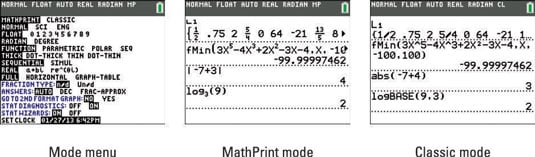## MathPrint mode versus Classic mode on the TI-84 Plus

The first choice on the Mode menu will have a big impact on the way your calculator displays expressions and answers. MathPrint is the default mode.

• MathPrint mode:

Fractions display like fractions, exponents look like exponents, text doesn’t wrap to the next line, and templates make it easier to enter commands. See the second screen.

• Classic mode:

Fractions use a forward slash (/) symbol, most exponents aren’t elevated, text wraps to the next line, and templates aren’t available. See the third screen.

One item in each row of this menu must be selected. Here are your choices:

• Normal, Sci, or Eng:

This setting controls how numbers are displayed on the calculator. In Normal mode, the calculator displays a number in the usual numeric fashion that you used in elementary school — provided it can display it using no more than ten digits. If the number requires more than ten digits, the calculator displays it using scientific notation.

In Scientific (Sci) mode, numbers are displayed using scientific notation; and in Engineering (Eng) mode, numbers are displayed in engineering notation. In the following figure, the first answer is displayed in normal notation, the second in scientific notation, and the third in engineering notation.

In scientific and engineering notation, the calculator uses En to denote multiplication by 10n.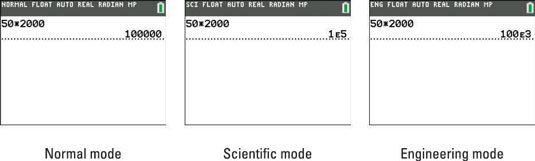• Float 0123456789:

Select Float if you want the calculator to display as many digits as possible. Select 0 if you want all numbers rounded to an integer. If you’re dealing with money, select 2 so that all numbers will be rounded to two decimal places. Selecting 5 rounds all numbers to five decimal places, and, well, you get the idea.

If you select Radian, all angles entered in the calculator are interpreted as being in radian measure; all angular answers given by the calculator will also be in radian measure. Similarly, if you select Degree, any angle you enter must be in degree measure, and any angular answer given by the calculator is also in degree measure.

• Function, Parametric, Polar, or Seq:

This setting tells the calculator what type of functions you plan to graph. Select Function to graph plain old vanilla functions in the form y = f(x). Select Parametric to graph parametric equations; Polar to graph polar equations; and Seq to graph sequences. (Sequences are also called iterative equations.)

• Thick, Dot–Thick, Thin, or Dot–Thin:

In Dot–Thick or Dot–Thin mode, the calculator produces a graph by plotting only the points it calculates. In Thick or Thin mode, the calculator joins consecutively plotted points with a line. Thick or Thin has to do with the thickness of the line style in the Y = editor.

If you want to quickly change the line styles of all of your functions at once, choose Thick, Dot–Thick, Thin, or Dot–Thin.

• Sequential or Simul:

In Sequential mode, the calculator completes the graph of one function before it graphs the next function. In Simultaneous (Simul) mode, the calculator graphs all functions at the same time. It does so by plotting the values of all functions for one value of the independent variable, and then plotting the values of all functions for the next value of the independent variable.

Simul mode is useful if you want to see whether two functions intersect at the same value of the independent variable. You have to watch the functions as they are graphed in order to see if this happens.

• Real,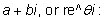If you’re dealing with only real numbers, select the Real mode. If you’re dealing with complex numbers, select a + bi if you want the complex numbers displayed in rectangular form. If you want complex numbers displayed in polar form, select the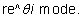• Full, Horizontal, or Graph-Table:

The Full screen mode displays the screen as you see it when you turn the calculator on. The other screen modes are split-screens. The Horizontal mode is for when you want to display a graph and the Y= editor or the Home screen at the same time. Use the Graph-Table mode when you want to display a graph and a table at the same time.

• Fraction Type: n/d or Un/d:

The results display as simple fractions or mixed numbers.

Changing this setting affects how the answers are displayed. Choosing Auto displays answers in a similar form as the input. Dec displays answers in decimal form. Frac-Approx displays answers in fraction form when possible.

• Go to 2nd Format Graph: NO, YES:

Choosing Yes redirects you to the Format Graph screen. Alternatively, you can press [2nd][ZOOM].

• Stat Diagnostics: OFF, ON:

Turn this ON so that r and r2 display when you run a regression.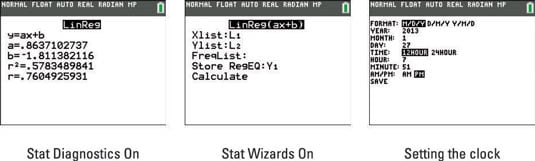• Stat Wizards: ON, OFF:

If you have this set to ON, an input screen provides syntax help for entering the proper syntax of certain statistical commands.

• Set Clock:

This is where you set the clock on the TI-84 Plus family of calculators. To do this, use the arrow keys to place the cursor on the SET CLOCK option and press [ENTER]. You see the third screen. You use the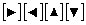keys to move from item to item. To select items in the first, fifth, and eighth rows, place the cursor on the desired item and press [ENTER] to highlight that item. To enter numbers in the other options, edit the existing number or press [CLEAR] and use the keypad to enter a new number. When you’re finished setting the clock, save your settings by placing the cursor on SAVE and pressing [ENTER].